### DS4420: Exercise on Dimensionality Reduction via PCA¶

In general dimensionality reduction may be viewed as encoding data $x$ into latent (lower dimensional) representations $z$ that one can then use to recover, noisily, the original $x$'s.

One may view this as an encoding-decoding process, where we encode $x$ into $z$, and then decode $z$ into a reconstruction $\tilde{x}$. We saw that if our objective is to capture as much variance as possible in the compressed space, then we can use eigenvectors; namely the top $m$ eigenvectors (ranked by corresponding eigenvalues) may be used to create an $m$ dimensional representation of $x$. In this exercise, we see this in practice.

In :
import numpy as np
import matplotlib.pyplot as plt

import torch
# conda install -c pytorch torchvision
import torchvision

# note: if you cannot get torchvision installed
# using the above sequence, you can resort to
# the colab version here:
# the notebook to blackboard when complete.
fMNIST = torchvision.datasets.FashionMNIST(
root = './data/FashionMNIST',
train = True,

In :
fMNIST.data.shape

Out:
torch.Size([60000, 28, 28])
In :
from IPython.display import Image
from matplotlib.pyplot import imshow
%matplotlib inline
imshow(np.asarray(fMNIST.data), cmap='gray')

Out:
<matplotlib.image.AxesImage at 0x7ff8fa4edc50>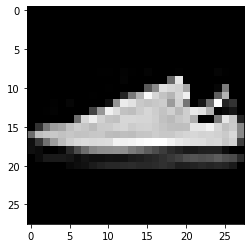In :
X = fMNIST.data
X = np.array([x_i.flatten().numpy() for x_i in X])
X = X / 255 # normalize
X.shape

Out:
(60000, 784)

### TODO 1¶

Zero-mean $X$, and then construct the covariance matrix $S = X^t X$. What is the shape of $S$?

In :
X_mu = X.mean(axis=0)
X = X - X_mu # zero-mean
S = (X.transpose() @ X)
S.shape

Out:
(784, 784)

### TODO 2¶

Use numpy.linalg.eigh to retrieve the top eigenvectors and eigenvalues of $S$. Then, take the top $M$ of these -- say $M=50$ -- and project $X$ into $Z$. How much variance is captured with this value of $M$?

In :
eigvals, eigvecs = np.linalg.eigh(S)

In :
M = 50
B = eigvecs[:, -M:]
B.shape

Out:
(784, 50)
In :
Z = X @ B
Z.shape

Out:
(60000, 50)
In :
sum(eigvals)

Out:
4092975.6596677555
In :
var_captured = np.sum(eigvals[-M:])/np.sum(eigvals)
print("{} components captures {}% of the variance".format(M, var_captured))

50 components captures 0.8626917002845209% of the variance


### TODO 3¶

Plot the variance captured as a function of number of components. Remember; this is given by the sum of the first $k$ eigenvalues over the total sum of the eigenvalues. Based on this plot, what do you think is a 'good' number of components to pick?

In :
total_var = np.sum(eigvals)
component_range = list(range(S.shape))
vars_captured = np.array([np.sum(eigvals[-(k+1):])/total_var for k in component_range])
plt.plot(component_range, vars_captured)

Out:
[<matplotlib.lines.Line2D at 0x7f81a2b4a390>]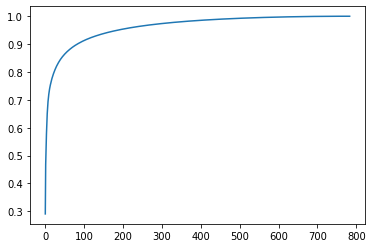### TODO 4¶

Now "decode" an image back from $Z$ to $\tilde{X}$. Visualize it (you can follow the above code, using imshow).

In :
X_tilde = Z @ B.transpose()
X_tilde.shape

Out:
(60000, 784)
In :
imshow(np.asarray(X_tilde+X_mu).reshape((28,28)), cmap='gray')

Out:
<matplotlib.image.AxesImage at 0x7f81c0469be0>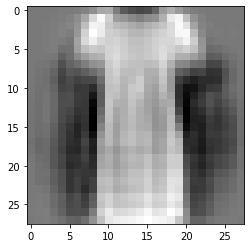In :
imshow(np.asarray(X + X_mu).reshape(28,28), cmap='gray')

Out:
<matplotlib.image.AxesImage at 0x7f81e404dc88>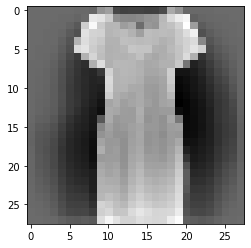### Bonus 1: Scatter two-dimensional representation of two item types (classes).¶

Do they separate well in this reduced space?

In :
y = fMNIST.train_labels.numpy()
#subset_indices = y[(y==1) | (y==7)]#np.argwhere((y==2 )|| (y==3))
ones = np.argwhere(y==1).flatten()
twos = np.argwhere(y==7).flatten()

In :
Z1 = Z[ones]
Z2 = Z[twos]
Zc = np.concatenate((Z1, Z2))
colors = ["blue"] * Z1.shape + ["red"] * Z2.shape

In :
Zc.shape

Out:
(12000, 50)
In :
plt.clf()
%matplotlib inline
plt.scatter(Zc[:,0], Zc[:,1], c=colors, alpha=.1)

Out:
<matplotlib.collections.PathCollection at 0x7f81b1111470>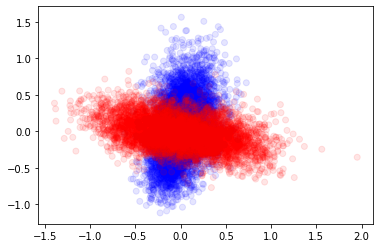### Bonus 2: Fun with Probabilistic PCA¶

In the MML reading, they derived that the posterior distribution over $z$ (the latent representation) given $x$ is:

$\mathcal{N}(m, C)$

Where:

$m = B^T (B B^T + \sigma^2I)^{-1} (x-u)$

$C = I - B^T(BB^T + \sigma^2I)^{-1}B$

In general we will assume $\sigma^2$ is known.

In :
def sample_from_posterior_given_x(x, var=0.1, samples=10):
B_term = B.transpose() @ np.linalg.inv((B @ B.transpose() + var * np.eye(B.shape)))
m = B_term @ (x - X_mu)
C = np.eye(B.shape) - B_term @ B
samples = np.array([np.random.multivariate_normal(m, C) for _ in range(samples)])
return samples

In :
samples = sample_from_posterior_given_x(X[1,:], var=1)

In :
decoded_samples = samples @ B.transpose()
decoded_samples.shape

Out:
(10, 784)
In :
imshow(np.asarray(X + X_mu).reshape(28,28), cmap='gray')

Out:
<matplotlib.image.AxesImage at 0x7f818113b1d0>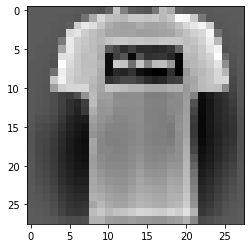In :
imshow(np.asarray(decoded_samples + X_mu).reshape(28,28), cmap='gray')

Out:
<matplotlib.image.AxesImage at 0x7f8180335470>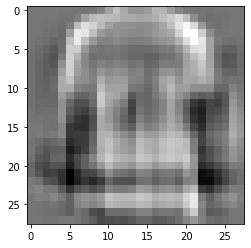In [ ]: